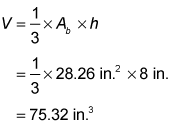##### Basic Math & Pre-Algebra Workbook For Dummies with Online Practice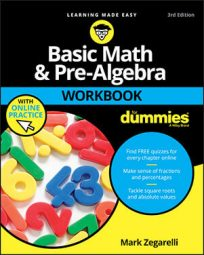The formula for the volume of a pyramid is the same as for the volume of a cone. In this formula, Ab is the area of the base, and h is the height: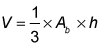## Practice questions

1. Find the volume of a pyramid with a square base whose side is 10 inches and with a height of 6 inches.
2. Find the approximate volume of a cone whose base has a radius of 3 inches and whose height is 8 inches.

1. 200 cubic inches

First, find the area of the base using the formula for the area of a square:

Ab = s2 = (10 in.)2 = 100 in.2

Now plug this result into the formula for the volume of a pyramid: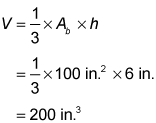2. Approximately 75.36 cubic inches

Use the area formula for a circle to find the area of the base: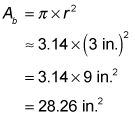Plug this result into the formula for the volume of a cone: